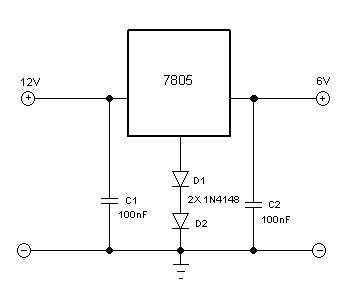# Converting 5V 7805 Linear Voltage Regulator to Produce 6.4V Output

Making a linear voltage regulator using 78XX integrated circuit series is very easy, it needs only one or two additional components to make the integrated circuit chip works. The problem arises when we need a custom voltage specs for the output, since they come in discrete fixed voltage variation (specified by XX parameter printed in the body of the IC package). In the following circuit  (Figure 1) we present a small  modification to rise up the output voltage, by adding two silicone diodes.Figure 1. The Schematic Diagram of Modified 7805 Linear Voltage Regulator Circuit to Produce 6.4V Output

The IC is connected to the supply voltage at pin 1, two diodes (in series) at pin2, and the output at pin 3. The diodes add small offset about 1.4V (0,7V per silicon diode) at the output. If we need 5.7V at the output then we need only one diode (not two diodes in series). It works since the integrated circuit regulate the output output at the specified voltage (5V) in relation to the ground reference (pin 2). We can use other diode such as Zener diode to get different output voltage specs. Connect the diode in the opposite way of regular 1N4148 diode,  since a Zener diode will work at its breakdown voltage (while regular diode works in its forward bias mode). See the circuit works in the Youtube video below.